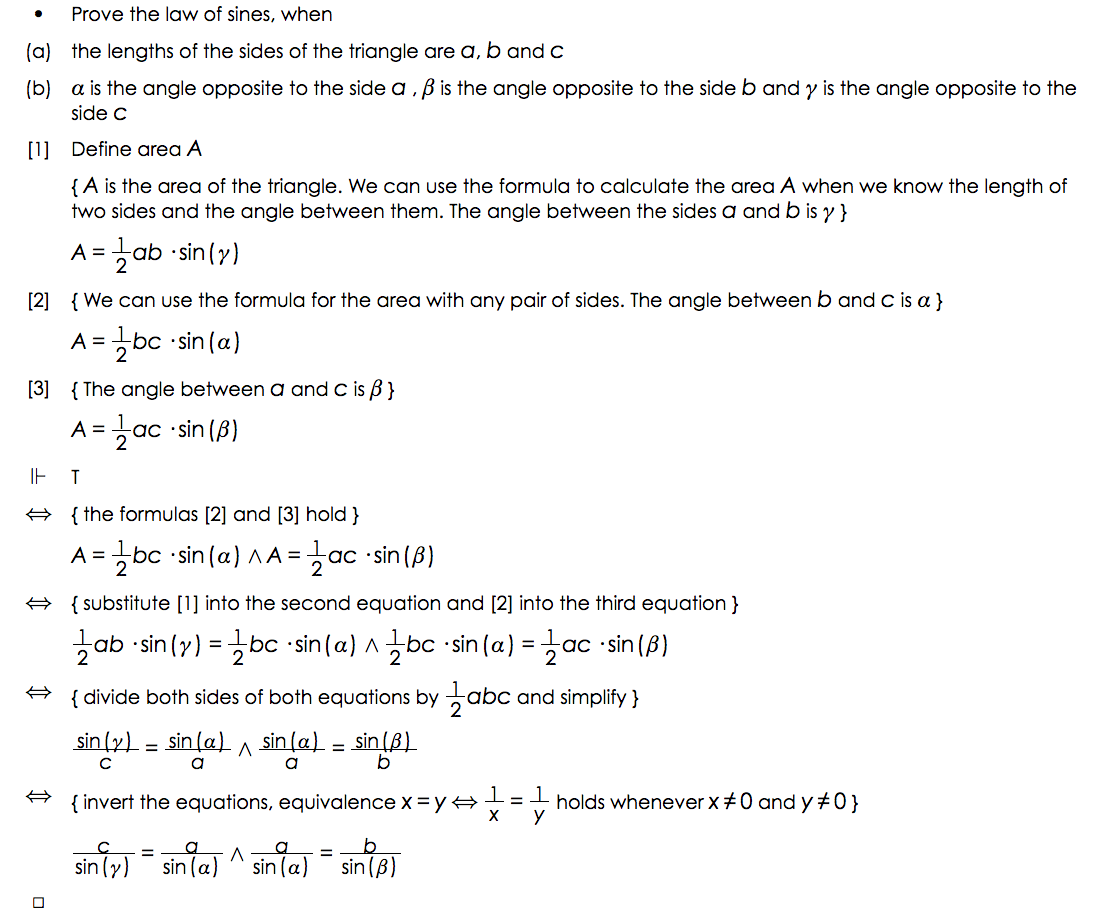# eMath Editor

### Editor for Structured Derivations

The SD editor is a syntax-oriented editor for writing structured derivations. This means that the editor guides the user into creating a structured derivation with the correct overall syntax.  The editor supports standard mathematical notation, both for creating mathematical formulas and for editing these.

### Constructing Structured Derivations

The eMath editor is designed to take care of the formatting so that you can concentrate on the mathematical reasoning itself.

The editor shows you the editing operations that are allowed at each place in the derivation. A derivation can be edited at any place, adding derivations steps, removing derivation steps, and editing a derivation step.

The editor uses standard mathematical notation in the derivation. Mathematical symbols can be written using LaTeX shortcuts or picked from a symbol palette.

In a calculation step, the editor first creates a copy of the previous term. This makes it easy to make small changes in large formulas, and reduces typing errors in the derivation.

### Constructing Structured Derivations

The eMath editor is designed to take care of the formatting so that you can concentrate on the mathematical reasoning itself.

The editor shows you the editing operations that are allowed at each place in the derivation. A derivation can be edited at any place, adding derivations steps, removing derivation steps, and editing a derivation step.

The editor uses standard mathematical notation in the derivation. Mathematical symbols can be written using LaTeX shortcuts or picked from a symbol palette.

In a calculation step, the editor first creates a copy of the previous term. This makes it easy to make small changes in large formulas, and reduces typing errors in the derivation.

### Advantages of Using the eMath Editor

The eMath editor enforces  correct syntax for structured derivations and uses standard mathematical (wysiwyg) notation. It can be used for derivations in any area of mathematics.

The editor gives complete freedom for how to write mathematical propositions, formulas, and justifications. It allows for changes at any place in the derivation:  adding, deleting and editing assumptions, calculation steps, facts and definitions.

The eMath editor can selectively show and hide nested derivations. Hiding the nested derivations allows you to see the overall argument, showing a nested derivation allows you to focus on details.

The eMath editor has a plug in for automatically checking the correctness of the derivation.